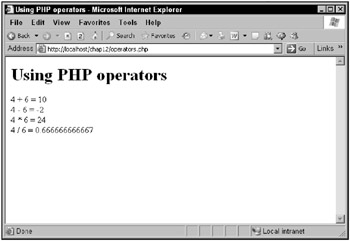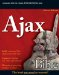There are many operators in PHP, and most of them do the same thing they do in JavaScript. Following is a list of the PHP operators:

• new

• [

• !~++--

• */%

• +-.

• == !=

• &

• |

• &&

• ||

• ?:

• =+=-=*=/=.=%=&=|=^=<<=>>=

The example, operators.php, puts some of these operators to work:

<html>     <head>         <title>             Using PHP operators         </title>     </head>          <body>         <h1>            Using PHP operators         </h1>         <?             echo "4 + 6 = ", 4 + 6, "<br>";             echo "4 - 6 = ", 4 - 6, "<br>";             echo "4 * 6 = ", 4 * 6, "<br>";             echo "4 / 6 = ", 4 / 6, "<br>";         ?>     </body> </html>

The results of operators.php are shown in Figure 12.9, where you can see that the PHP operators have done what they should.Figure 12.9: Using PHP operatorsAjax Bible
ISBN: 0470102632
EAN: 2147483647
Year: 2004
Pages: 169

Similar book on Amazon